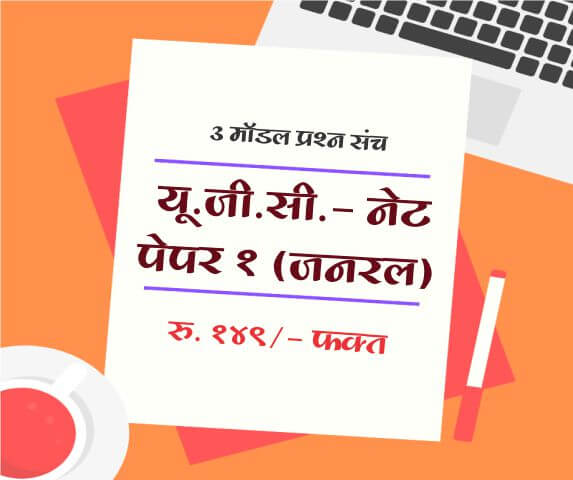# Simple Interest Calculator: Aptitude Test

1 What is Simple interest?

The percentage of the principal that is paid over a certain period of time is called the interest rate. Interest is typically calculated over principal amount (Base Amount).

Simple Interest is considered when time period is generally less than a year. Simple interest is calculated only on principal amount and Simple interest formula is as follows

$I=\frac{\mathrm{\left( P × n × r \right)}}{100}$
Where
I = Interest Amount
P = Principal Amount
n = Time period
r = Rate of interest

Candidates preparing for following competitive exams can utilize Aptitude section to improve their skills.

• Bank Competitive Exam
• MPSC Competitive Exams
• SSC Competitive Exams
• L.I.C/ G. I.C Competitive Exams
• Railway Competitive Exam
• University Grants Commission (UGC)
• NET/ SET Competitive Exam
• Common Aptitude Test (CAT)
• Career Aptitude Test (IT Companies)
2 How to calculate Interest earned?
3 How to calculate Principal Amount?
4 How to calculate Rate of Interest?
5 How to calculate time period?
6 How to calculate Amount accrued i.e. principal + interest earned?
Simple Interest Calculator

 Calculate Principal (P) Interest (I) Period (n) Rate of Interest (r) Principal + Interest (P + I)
 Interest Amount (I) Period (n) Rate of Interest (r) (% per year)

 Name : Comment :

Subscribe to get latest updates about Exam Schedules, Government Jobs, General Knowledge & Current Affairs and Many more on Whatsapp!###### Aptitude

Full Length Mock Tests
Timer Based Exams
Instant Result and assesment
Detailed analasys of Result##### UGC NET SET Paper 1 Mock Tests

UGC-NET / SET Paper 1 mock test

3 Mock Tests, 150 Questions with Explanations

Rs: 149 Only/-##### LAW MH-CET 2019 (3 YEAR COURSE)

Mock test for MH CET Law 2019 for three year law programme

3 Mock Tests, 450 Questions with Explanations

Rs: 249 Only/-##### यू. जी. सी. - नेट पेपर १ (जनरल)

यू. जी. सी. - नेट पेपर १ (अनिवार्य) सामान्य प्रश्न हिंदीमें

3 Mock Tests, 150 Questions with Explanations

Rs: 149 Only/-